## 升级给力 2017款宝马3系售28.8-48.69万

2016年09月19日 11:01 来源：www.chextx.com 超过：次关注

9月19日，2017款宝马3系于全国正式上市，售价区间为28.8-48.69万元。新车顺应市场需求，在长轴距系列中推出了犀利动感的M运动型车型，并置入了系列智能化新配置将车型进行了升级。随之，具体车型命名也进行了更改，以318取代316，330取代328，335进阶为340。

 2017款宝马3系 官方指导售价 车型 售价（万元） 318i 时尚型 28.83 18Li 时尚型 30.99 320i M运动型 32.00 340i xDrive M运动型 68.43 20Li 时尚型 32.53 20Li xDrive 时尚型 34.99 320Li M运动型 35.39 320Li 豪华设计套装 37.99 330i M运动型 45.23 30Li M运动型 43.09 330Li xDrive豪华设计套装 48.69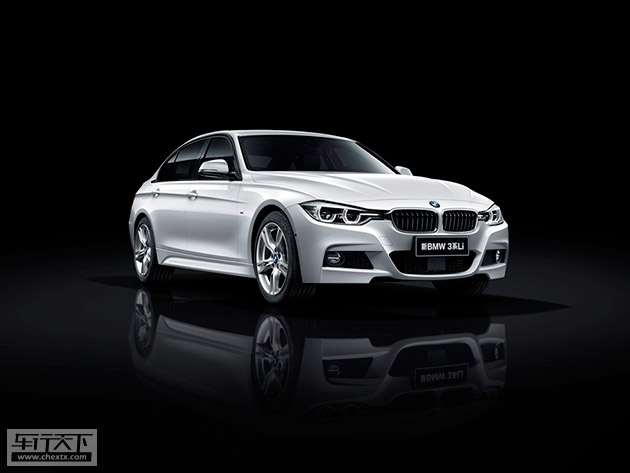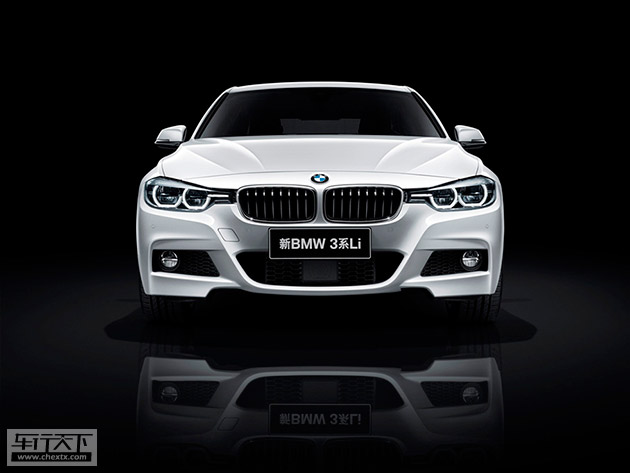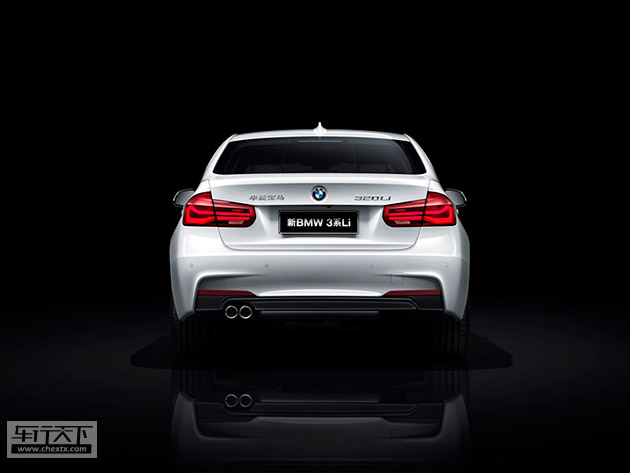首先，让我们来说说全新长轴距M运动型车型。没想到，如今备受欢迎的M运动套件现已扩展至BMW 3系长轴距车型。据悉，在长轴距车型上，M运动套件拥有多达12项专属装备，无论外观还是内饰都属性鲜明。外观上包括独特的M 18英寸轮圈、熏黑双肾进气格栅、M空气动力学套件等典型配置，并在车身上带有M标识。车内则提供有M门槛饰条、M专属仪表盘、M专属皮革方向盘以及M专属钥匙。除此之外，所有M运动型车型更标配带换挡拨片的8速运动型手自一体变速箱。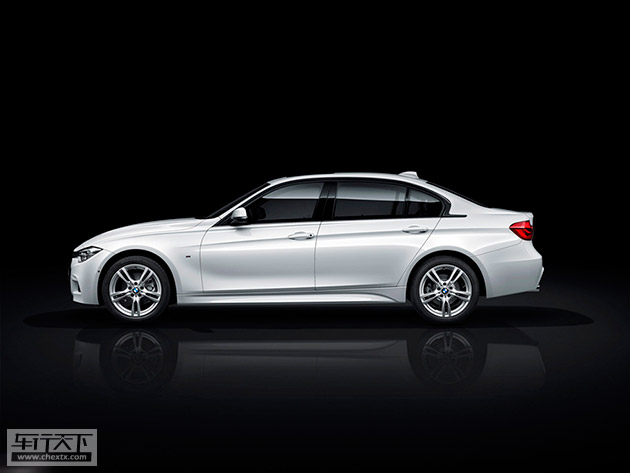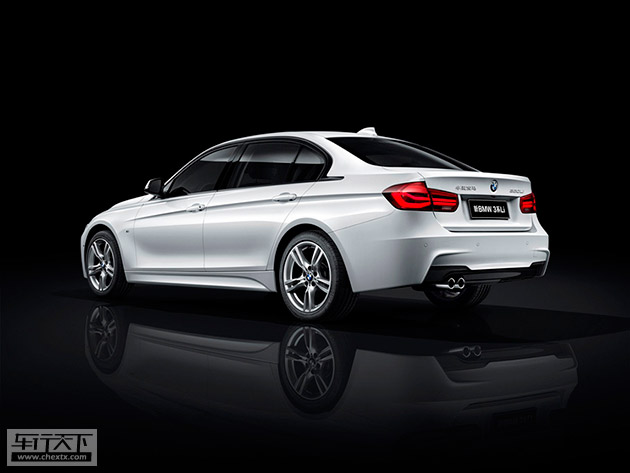不仅如此，如果你想要再进一步突显运动个性，还可选择M Performance高性能系列配件。

其次，换装的全新一代涡轮增压发动机,优势也是更加明显。据了解，2017款宝马3系已全部更新为宝马最新一代1.5T三缸、2.0T四缸与3.0T六缸涡轮增压汽油发动机。另外，作为同级唯一配备8速手自一体变速箱的新款3系，其变速箱也同步进行了升级，传动系统最大可承受500Nm的扭矩负荷，性能向旗舰车型7系看齐。以2017款330Li M运动型为例，在保持0-100km/h加速6.3S成绩的前提下，油耗仅为6.3L，较现款328Li油耗降幅多达12.5%。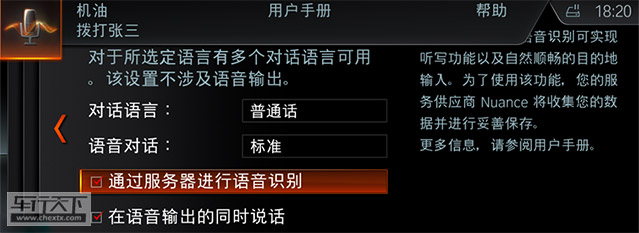“自然语音识别系统”与互联智能的搭载，是新款车型的第三大看点。“自然语音识别系统”通过日常用语，用户即可控制电话、导航、娱乐和短信等相关功能，实现最简单直接有效的人车互动。其最大亮点是一步到位的语音搜索导航目的地。如果使用传统的语音指令，用户需要按顺序层级分段说出地址信息，而使用这套新系统时，一句“我要去大悦城”即解决问题。另外，像“该加油了”这样的指令同样能找到驾驶者需要的兴趣点。在短信听写方面，用户可以直接陈述“给张三发短信，告诉他‘今晚一起吃饭’”，或者随性地告诉系统“把‘今晚’改成‘明晚’”。各种方便的日常用语都可以让人车进行顺畅地沟通。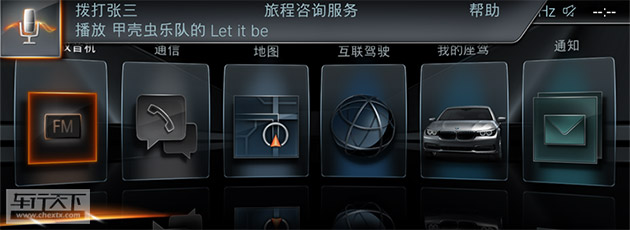此外，应用于旗舰车型7系的新一代iDrive 人机交互界面也引入到了2017款3系上。

最后，那些升级了的配置也不得不提一下。2017款3系在配置上继续丰富，比如M运动型配备了全彩平视显示系统，长轴距车型还标配有防眩目内外后视镜、外后视镜电动折叠等。

作为改款车型，2017款宝马3系融入了诸多新的智能化技术与配置，动力性能上也得到了最新升级，产品力无疑得到了进一步增强。

#### 相关文章

﻿
• 快速找车
• 选择品牌
• 选择品牌
• A  奥迪
• A  阿斯顿·马丁
• A  阿尔法·罗密欧
• B  宝沃
• B  布加迪
• B  巴博斯
• B  保时捷
• B  宾利
• B  奔驰
• B  宝马
• B  本田
• B  别克
• B  标致
• B  比亚迪
• B  宝骏
• B  北汽制造
• B  北汽新能源
• B  北汽幻速
• B  北汽威旺
• B  北京汽车
• B  奔腾
• B  北汽绅宝
• B  北汽昌河
• C  长安欧尚
• C  长安
• C  长安凯程
• C  长城
• D  大众
• D  道奇
• D  DS
• D  东南
• D  东风风神
• D  东风风行
• D  东风小康
• D  东风风度
• D  东风
• F  福特
• F  丰田
• F  菲亚特
• F  法拉利
• F  福田
• F  福迪
• F  福汽启腾
• G  观致
• G  广汽传祺
• G  广汽吉奥
• G  GMC
• H  红旗
• H  汉腾汽车
• H  哈弗
• H  哈飞
• H  海格
• H  海马
• H  华颂
• H  黄海
• H  华泰
• H  恒天
• J  几何汽车
• J  捷达
• J  吉利汽车
• J  捷豹
• J  Jeep
• J  江淮
• J  江铃
• J  金杯
• J  九龙
• J  金旅
• K  凯翼
• K  凯迪拉克
• K  克莱斯勒
• K  科尼塞克
• K  卡威
• K  开瑞
• L  路虎
• L  林肯
• L  劳斯莱斯
• L  兰博基尼
• L  雷克萨斯
• L  铃木
• L  领克
• L  雷诺
• L  理念
• L  力帆
• L  莲花汽车
• L  猎豹
• L  路特斯
• L  陆风
• M  马自达
• M  MG
• M  MINI
• M  玛莎拉蒂
• M  摩根
• M  迈凯轮
• N  纳智捷
• O  欧拉
• O  欧宝
• O  讴歌
• O  欧朗
• Q  奇瑞
• Q  起亚
• Q  启辰
• R  日产
• R  荣威
• R  瑞麒
• S  SERES赛力斯
• S  三菱
• S  斯威汽车
• S  萨博
• S  smart
• S  斯柯达
• S  斯巴鲁
• S  思铭
• S  双龙
• S  上汽大通
• S  双环
• T  特斯拉
• T  腾势
• W  蔚来
• W  沃尔沃
• W  WEY
• W  五菱汽车
• W  五十铃
• W  威兹曼
• W  威麟
• X  现代
• X  雪佛兰
• X  星途
• X  雪铁龙
• X  小鹏汽车
• X  西雅特
• Y  一汽
• Y  英菲尼迪
• Y  英致
• Y  依维柯
• Y  野马汽车
• Y  永源
• Z  众泰
• Z  中华
• Z  中兴
• Z  知豆
• 选择车系
• 选择车系
• 车型对比
• 选择品牌
• 选择品牌
• A  奥迪
• A  阿斯顿·马丁
• A  阿尔法·罗密欧
• B  宝沃
• B  布加迪
• B  巴博斯
• B  保时捷
• B  宾利
• B  奔驰
• B  宝马
• B  本田
• B  别克
• B  标致
• B  比亚迪
• B  宝骏
• B  北汽制造
• B  北汽新能源
• B  北汽幻速
• B  北汽威旺
• B  北京汽车
• B  奔腾
• B  北汽绅宝
• B  北汽昌河
• C  长安欧尚
• C  长安
• C  长安凯程
• C  长城
• D  大众
• D  道奇
• D  DS
• D  东南
• D  东风风神
• D  东风风行
• D  东风小康
• D  东风风度
• D  东风
• F  福特
• F  丰田
• F  菲亚特
• F  法拉利
• F  福田
• F  福迪
• F  福汽启腾
• G  观致
• G  广汽传祺
• G  广汽吉奥
• G  GMC
• H  红旗
• H  汉腾汽车
• H  哈弗
• H  哈飞
• H  海格
• H  海马
• H  华颂
• H  黄海
• H  华泰
• H  恒天
• J  几何汽车
• J  捷达
• J  吉利汽车
• J  捷豹
• J  Jeep
• J  江淮
• J  江铃
• J  金杯
• J  九龙
• J  金旅
• K  凯翼
• K  凯迪拉克
• K  克莱斯勒
• K  科尼塞克
• K  卡威
• K  开瑞
• L  路虎
• L  林肯
• L  劳斯莱斯
• L  兰博基尼
• L  雷克萨斯
• L  铃木
• L  领克
• L  雷诺
• L  理念
• L  力帆
• L  莲花汽车
• L  猎豹
• L  路特斯
• L  陆风
• M  马自达
• M  MG
• M  MINI
• M  玛莎拉蒂
• M  摩根
• M  迈凯轮
• N  纳智捷
• O  欧拉
• O  欧宝
• O  讴歌
• O  欧朗
• Q  奇瑞
• Q  起亚
• Q  启辰
• R  日产
• R  荣威
• R  瑞麒
• S  SERES赛力斯
• S  三菱
• S  斯威汽车
• S  萨博
• S  smart
• S  斯柯达
• S  斯巴鲁
• S  思铭
• S  双龙
• S  上汽大通
• S  双环
• T  特斯拉
• T  腾势
• W  蔚来
• W  沃尔沃
• W  WEY
• W  五菱汽车
• W  五十铃
• W  威兹曼
• W  威麟
• X  现代
• X  雪佛兰
• X  星途
• X  雪铁龙
• X  小鹏汽车
• X  西雅特
• Y  一汽
• Y  英菲尼迪
• Y  英致
• Y  依维柯
• Y  野马汽车
• Y  永源
• Z  众泰
• Z  中华
• Z  中兴
• Z  知豆
• 选择车系
• 选择车系
• 选择车型
• 选择车型
• 意见反馈